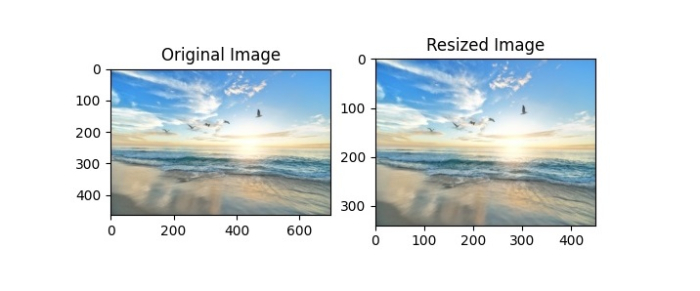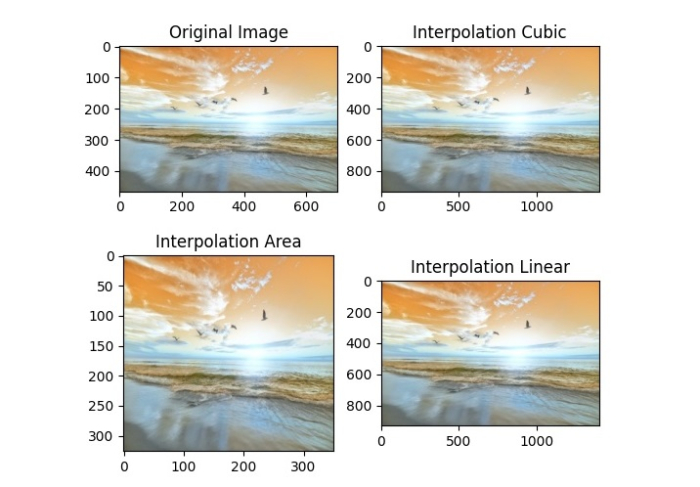# How to resize an image in OpenCV using Python?

OpenCV provides the function cv2.resize() to resize an image. Resizing in OpenCV is referred to as scaling. We can resize an image by specifying the image size or scaling factor. The aspect ratio is preserved when we specify the scaling factor.

There are different interpolation methods used in cv2.resize() function −

• cv2.INTER_AREA − Used for shrinking an image.

• cv2.INTER_CUBIC − It’s slow, used for zooming.

• cv2.INTER_LINEAR − Used for zooming. It is default for all resizing purposes.

## Steps

You can use the following steps to resize an image −

Import the required libraries. In all the following Python examples, the required Python libraries are OpenCV and Matplotlib. Make sure you have already installed them.

import cv2
import matplotlib.pyplot as plt


Read an image using cv2.imread() function. Specify the full image path with image types (.jpg or .png).

img = cv2.imread('birds.jpg')


Resize the image passing the new_size or the scaling factors fx and fy and the interpolation. fx and fy are scale factors to width and height respectively.

resize_img = cv2.resize(img, new_size)
resize_img = cv2.resize(img,(0, 0),fx=0.5, fy=0.7, interpolation = cv2.INTER_AREA)


Display the resized image(s).

plt.imshow(resize_img)


Let's understand the different image resizing options with the help of some Python examples.

We will use this image as the input file in the following examples −## Example 1

In the following Python program, we resize the input image to new_size = 450, 340). Here width=450 and height=340.

import cv2
import matplotlib.pyplot as plt

h, w, c = img.shape
print(f"Height and width of original image: {h}, {w}" )

# resize the image
new_size = (450, 340) # new_size=(width, height)
print(f"New height and width: {new_size}, {new_size}" )
resize_img = cv2.resize(img, new_size)

# Convert the images from BGR to RGB
img = cv2.cvtColor(img, cv2.COLOR_BGR2RGB)
resize_img = cv2.cvtColor(resize_img, cv2.COLOR_BGR2RGB)

plt.subplot(121),plt.imshow(img), plt.title("Original Image")
plt.subplot(122), plt.imshow(resize_img), plt.title("Resized Image")
plt.show()


## Output

When you run the above program, it will produce the following output −

Height and width of original image: 465, 700
New height and width of original image: 340, 450

And it will display the following output window showing the original and resized images.## Example 2

In the Python program below, we resize the input image using different scaling factors and interpolations.

# import the required libraries
import cv2
import matplotlib.pyplot as plt

# resize the image using different interpolations
resize_cubic = cv2.resize(img,(0, 0),fx=2, fy=2, interpolation = cv2.INTER_CUBIC)
resize_area = cv2.resize(img,(0, 0),fx=0.5, fy=0.7, interpolation = cv2.INTER_AREA)
resize_linear = cv2.resize(img,(0, 0),fx=2, fy=2, interpolation = cv2.INTER_LINEAR)

# display the original and resized images
plt.subplot(221),plt.imshow(img), plt.title("Original Image")
plt.subplot(222), plt.imshow(resize_cubic), plt.title("Interpolation Cubic")
plt.subplot(223), plt.imshow(resize_area), plt.title("Interpolation Area")
plt.subplot(224), plt.imshow(resize_linear), plt.title("Interpolation Linear")
plt.show()


## Output

When you run the above program, it will produce the following output−